# Riesz-Fischer theorem

(diff) ← Older revision | Latest revision (diff) | Newer revision → (diff)
A theorem establishing a relationship between the spaces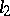and: If a system of functionsis orthonormal on the interval(cf. Orthonormal system) and a sequence of numbers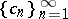is such that(that is,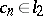), then there exists a functionfor which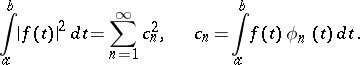Moreover, the functionis unique as an element of the space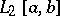, i.e. up to its values on a set of Lebesgue measure zero. In particular, if the orthonormal system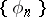is closed (complete, cf. Complete system of functions) in, then, using the Riesz–Fischer theorem, one gets that the spacesandare isomorphic and isometric.